# 4 integers are enough to write a Snake Game

… actually you can use only 2, but this will make your life a little more miserable.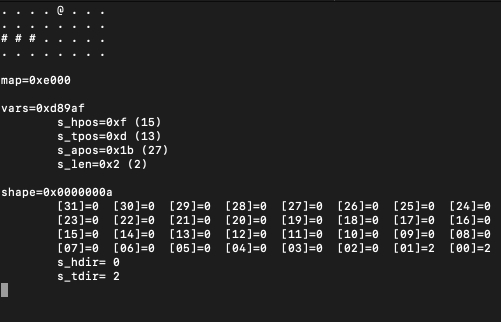After not implementing a game of snake in ages, I’ve decided to do my best today, but with some strange and absurd limitations in mind, you know, to spice up things:

• We will store the game map in a `uint32_t` where `1s` will form the reptile’s body. The map will contain `4x8` positions. Enough to have fun!
• We will keep another `uint64_t` as a directions array - this will be useful to move the snake around, while keeping its growing shape intact;
• We will squeeze another a few 5 bits values in an `uint32_t` to keep the positions of the `head`, the `tail`, the `apple`, and the (current) `length`. Any input from the keyboard will also be kept here (2 bits will be enough).
• We will keep an 8 bit (`uint8_t`) variable for looping.

Because there’s no standard C-way of interacting with the keyboard, I will have to rely on `curses`, so if you want to compile the program, make sure you have the lib installed on your system. If you’re using the right type of operating system, chances are it’s already there. If not you can certainly install it from your favorite package manager.

Unfortunately,`curses` uses additional memory by itself, but let’s be honest, hacking with arcane escape chars and low level system functions is not fun, and certainly not something that I am willing to try by myself. Yes, it’s cheating, and this article is a fraud!

Before continue reading (if you haven’t stopped by now), note that the code should be taken as a twisted joke, or as an exercise in minimalism, or as both, probably a joke. Because of the aforementioned limitations, we are going to write some nasty macros to perform bitwise operations, use global variables, reuse the same counter, etc. This is not a good example of readable or elegant code.

Everything is available on GitHub:

``````git clone git@github.com:nomemory/integers-snake.git
``````

To compile and run the program:

``````gcc -Wall snake.c -lcurses && ./a.out
``````

We will start by defining the 4 integers that will hold all our game data:

``````uint32_t map = ...;
uint32_t vars = ...;
uint64_t shape = ...;
int8_t i = ...;
``````

### `map`Permalink

`map` is what we are going to display on the screen. The `32` bits of `map` will form a `4x8` grid that will be rendered using curses: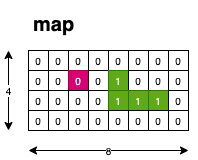To access the memory and set bits to zero or one, we can use the following macros:

``````#define s_is_set(b) ((map&(1<<(b)))!=0) // checks if the b bit from the map is set to 1
#define s_tog(b) (map^=(1<<(b))) // toggles the b bit of the map (currently not used)
#define s_set_0(b) (map&=~(1<<b)) // sets to 0 the b bit from the map
#define s_set_1(b) (map|=(1<<b)) // sets to 1 the b bit from the map
``````

### `vars`Permalink

`vars` is a `32` bits integer where will keep the following data: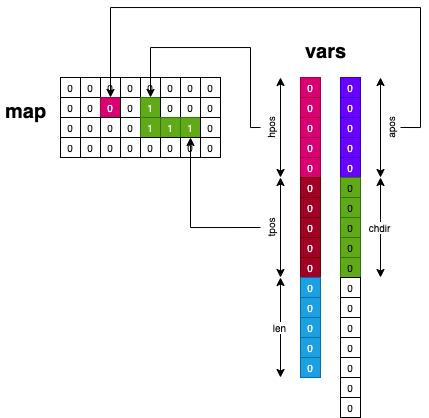• `hpos` (from bit `0` to `4`) represents the head position of the snake as an offset from the map’s LSB;
• `tpos` (from bit `5` to `9`) represents the tail position of the snake as an offset from the map’s LSB;
• `len` (from bit `10` to `14`) represents the length of the snake;
• `apos` (from bit `15` to `19`) represents the apple position as an offset from the map’s LSB;
• `chdir` (from bit `20` to `21`) represents the last key pressed, `2` bits are enough, because only arrows are registered;
• the remaining bits are not used;

To access `hpos`, `tpos`, etc. we have defined the following macros:

``````#define s_mask(start,len) (s_ls_bits(len)<<(start))
#define s_prep(y,start,len) (((y)&s_ls_bits(len))<<(start))

// Gets the the 'len' number of bits, starting from position 'start' of 'y'
#define s_get(y,start,len) (((y)>>(start))&s_ls_bits(len))
// Sets the the 'len' number of bits, starting from position 'start' of 'y' to the value 'bf'

#define s_hpos s_get(vars,0,5) // gets the last 5 bits of 'vars', which corresponds to s_hpos
#define s_tpos s_get(vars,5,5) // sets the last 5 bits of 'vars', which corresonds to s_hpos
#define s_len s_get(vars,10,5)
#define s_apos s_get(vars,15,5)
#define s_chdir s_get(vars,20,2)
#define s_hpos_set(pos) s_set(vars,pos,0,5)
#define s_tpos_set(pos) s_set(vars,pos,5,5)
#define s_len_set(len) s_set(vars,len,10,5)
#define s_apos_set(app) s_set(vars,app,15,5)
#define s_chdir_set(cdir) s_set(vars,cdir,20,2)
#define s_len_inc s_len_set(s_len+1)
``````

### `shape`Permalink

`shape` keeps the directions for each cell of the snake. `2` bits per direction are enough, so we can keep a total of 32 directions: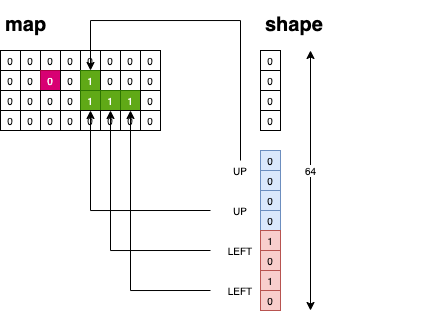The possible directions are mapped using the following macros:

``````#define SU 0 //UP
#define SD 1 //DOWN
#define SL 2 //LEFT
#define SR 3 //RIGHT
``````

Each time the snake moves inside the `map` grid, we cycle through the directions, with the following macros:

``````#define s_hdir ((shape>>(s_len*2)&3))
#define s_tdir (shape&3)
#define s_hdir_set(d) s_set(shape,d,s_len*2,2)
#define s_tdir_set(d) s_set(shape,d,0,2)
#define s_shape_rot(nd) do { shape>>=2; s_hdir_set(nd); } while(0);
#define s_shape_add(nd) do { s_len_inc; shape<<=2; s_tdir_set(nd); } while(0);
``````

When the snake moves, without eating an apple we call the `s_shape_rot` macro that removes the last direction, and pushes a new head (based on `s_chdir`).

In this regard, shape behaves like a queue: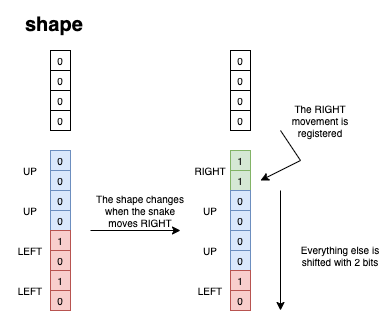When the snake moves and eats an apple we call `s_shape_add` that increases the length, and pushes a new tail `s_tdir`.

The game loop looks like this:

``````#define s_init do { srand(time(0)); initscr(); keypad(stdscr, TRUE); cbreak(); noecho(); } while(0);
#define s_exit(e) do { endwin(); exit(e); } while(0);
#define s_key_press(k1, k2) if (s_hdir==k2) break; s_chdir_set(k1); break;

int main(void) {
s_init; // initialize the curses context
rnd_apple(); // creates a random position for the apple
while(1) {
show_map(); // renders the map on screen
timeout(80); // getch() timeouts after waiting for user input
switch (getch()) {
case KEY_UP : { s_key_press(SU, SD) };
case KEY_DOWN : { s_key_press(SD, SU) };
case KEY_LEFT : { s_key_press(SL, SR) };
case KEY_RIGHT : { s_key_press(SR, SL) };
case 'q' : exit(0); // Quits the game
}
move_snake(); // The snake moves inside the grid
s_shape_rot(s_chdir); // The shape is getting updated
napms(200); // frame rate :))
}
s_exit(0); // games exits
}
``````

Each time a key is pressed `s_key_press` is expanded. This checks if the movement is possible, and then updates the `s_chdir` (using `s_chdir_set`).

The reason `s_key_press` has two input paramameters is to exclude the opposite direction. For example if the snake is currently moving to the RIGHT (`SR`), a `SL` is not possible, and thus we break the switch.

### The function that moves the snake:Permalink

`move_snake()` is where most of our logic is implemented:

``````#define s_next_l s_mask5(s_hpos+1)
// Check if a left movement is possible.
static void check_l() { if ((s_mod_p2(s_next_l,8) < s_mod_p2(s_hpos,8)) || s_is_set(s_next_l)) s_exit(-1); }
// Check if a right movement is possible.
static void check_r() { if ((s_mod_p2(s_next_r,8) > s_mod_p2(s_hpos,8)) || s_is_set(s_next_r)) s_exit(-1); }
// Check if a up movement is possible
static void check_u() { if ((s_next_u < s_hpos) || s_is_set(s_next_u)) s_exit(-1); }
// Check if a down movement is possible
static void check_d() { if ((s_next_d > s_hpos) || s_is_set(s_next_d)) s_exit(-1); }
static void move_snake() {
if (s_hdir==SL) { check_l(); s_hpos_set(s_hpos+1); }
else if (s_hdir==SR) { check_r(); s_hpos_set(s_hpos-1); }
else if (s_hdir==SU) { check_u(); s_hpos_set(s_hpos+8); }
else if (s_hdir==SD) { check_d(); s_hpos_set(s_hpos-8); }
// Sets the bit based on the current s_hdir and s_hpos
s_set_1(s_hpos);
// If an apple is ate
if (s_apos==s_hpos) {
// We generate another apple
rnd_apple();
// Append to the tail
// We stop clearning the tail bit
return;
}
// Clear the tail bit
s_set_0(s_tpos);
// Update the t_pos so we can clear the next tail bit when the snake moves
if (s_tdir==SL) { s_tpos_set(s_tpos+1); }
else if (s_tdir==SR) { s_tpos_set(s_tpos-1); }
else if (s_tdir==SU) { s_tpos_set(s_tpos+8); }
else if (s_tdir==SD) { s_tpos_set(s_tpos-8); }
}
``````

To validate if the snake can move or not in the grid, we’ve implemented the `check_*()` functions:

• `check_l()` - we check if the X coordinate of the snake (the modulo `%8` of the `s_hpos`) is bigger than the one from the previous position;
• `check_r()` - we check if the X coordinate of the snake (the modulo `%8` of the `s_hpos`) is smaller than the one from the previous position;
• `check_u()` and `check_d` work in the same way, they see if the by incrementing `s_hpos` it overflows. If it does, then it means we’ve exited the grid.

### The function that displays the snakePermalink

This is the last function we are going to implement:

``````static void show_map() {
clear();
i=32;
while(i-->0) {
// If the bit is an apple, we render the apple '@'
// We draw either the snake bit ('#') or the empty bit ('.')
// We construct the grid by inserting a new line
};
}
``````

After all the macros expands, the resulting code looks like this:

``````uint32_t map = 0x700;
uint32_t vars = 0x20090a;
uint64_t shape = 0x2a;
int8_t i = 0;
static void rnd_apple() {
i = (rand()&(32 -1));
while(((map&(1<<(i)))!=0)) i = (rand()&(32 -1));
(vars=((vars)&~(((1<<(5))-1)<<(15)))|(((i)&((1<<(5))-1))<<(15)));
}
static void show_map() {
wclear(stdscr);
i=32;
while(i-->0) {
if (!(i&(8 -1))) { waddch(stdscr,'\n'); }
};
}
static void check_l() { if ((((((((vars)>>(0))&((1<<(5))-1))+1)&0x1f)&(8 -1)) < ((((vars)>>(0))&((1<<(5))-1))&(8 -1))) || ((map&(1<<((((((vars)>>(0))&((1<<(5))-1))+1)&0x1f))))!=0)) do { endwin(); exit(-1); } while(0);; }
static void check_r() { if ((((((((vars)>>(0))&((1<<(5))-1))-1)&0x1f)&(8 -1)) > ((((vars)>>(0))&((1<<(5))-1))&(8 -1))) || ((map&(1<<((((((vars)>>(0))&((1<<(5))-1))-1)&0x1f))))!=0)) do { endwin(); exit(-1); } while(0);; }
static void check_u() { if (((((((vars)>>(0))&((1<<(5))-1))+8)&0x1f) < (((vars)>>(0))&((1<<(5))-1))) || ((map&(1<<((((((vars)>>(0))&((1<<(5))-1))+8)&0x1f))))!=0)) do { endwin(); exit(-1); } while(0);; }
static void check_d() { if (((((((vars)>>(0))&((1<<(5))-1))-8)&0x1f) > (((vars)>>(0))&((1<<(5))-1))) || ((map&(1<<((((((vars)>>(0))&((1<<(5))-1))-8)&0x1f))))!=0)) do { endwin(); exit(-1); } while(0);; }
static void move_snake() {
if (((shape>>((((vars)>>(10))&((1<<(5))-1))*2)&3))==2) { check_l(); (vars=((vars)&~(((1<<(5))-1)<<(0)))|((((((vars)>>(0))&((1<<(5))-1))+1)&((1<<(5))-1))<<(0))); }
else if (((shape>>((((vars)>>(10))&((1<<(5))-1))*2)&3))==3) { check_r(); (vars=((vars)&~(((1<<(5))-1)<<(0)))|((((((vars)>>(0))&((1<<(5))-1))-1)&((1<<(5))-1))<<(0))); }
else if (((shape>>((((vars)>>(10))&((1<<(5))-1))*2)&3))==0) { check_u(); (vars=((vars)&~(((1<<(5))-1)<<(0)))|((((((vars)>>(0))&((1<<(5))-1))+8)&((1<<(5))-1))<<(0))); }
else if (((shape>>((((vars)>>(10))&((1<<(5))-1))*2)&3))==1) { check_d(); (vars=((vars)&~(((1<<(5))-1)<<(0)))|((((((vars)>>(0))&((1<<(5))-1))-8)&((1<<(5))-1))<<(0))); }
(map|=(1<<(((vars)>>(0))&((1<<(5))-1))));
if ((((vars)>>(15))&((1<<(5))-1))==(((vars)>>(0))&((1<<(5))-1))) {
rnd_apple();
do { (vars=((vars)&~(((1<<(5))-1)<<(10)))|((((((vars)>>(10))&((1<<(5))-1))+1)&((1<<(5))-1))<<(10))); shape<<=2; (shape=((shape)&~(((1<<(2))-1)<<(0)))|((((shape&3))&((1<<(2))-1))<<(0))); } while(0);;
return;
}
(map&=~(1<<(((vars)>>(5))&((1<<(5))-1))));
if ((shape&3)==2) { (vars=((vars)&~(((1<<(5))-1)<<(5)))|((((((vars)>>(5))&((1<<(5))-1))+1)&((1<<(5))-1))<<(5))); }
else if ((shape&3)==3) { (vars=((vars)&~(((1<<(5))-1)<<(5)))|((((((vars)>>(5))&((1<<(5))-1))-1)&((1<<(5))-1))<<(5))); }
else if ((shape&3)==0) { (vars=((vars)&~(((1<<(5))-1)<<(5)))|((((((vars)>>(5))&((1<<(5))-1))+8)&((1<<(5))-1))<<(5))); }
else if ((shape&3)==1) { (vars=((vars)&~(((1<<(5))-1)<<(5)))|((((((vars)>>(5))&((1<<(5))-1))-8)&((1<<(5))-1))<<(5))); }
}

int main(void) {
do { srand(time(0)); initscr(); keypad(stdscr, 1); cbreak(); noecho(); } while(0);;
rnd_apple();
while(1) {
show_map();
wtimeout(stdscr,80);
switch (wgetch(stdscr)) {
case 0403 : { if (((shape>>((((vars)>>(10))&((1<<(5))-1))*2)&3))==1) break; (vars=((vars)&~(((1<<(2))-1)<<(20)))|(((0)&((1<<(2))-1))<<(20))); break; };
case 0402 : { if (((shape>>((((vars)>>(10))&((1<<(5))-1))*2)&3))==0) break; (vars=((vars)&~(((1<<(2))-1)<<(20)))|(((1)&((1<<(2))-1))<<(20))); break; };
case 0404 : { if (((shape>>((((vars)>>(10))&((1<<(5))-1))*2)&3))==3) break; (vars=((vars)&~(((1<<(2))-1)<<(20)))|(((2)&((1<<(2))-1))<<(20))); break; };
case 0405 : { if (((shape>>((((vars)>>(10))&((1<<(5))-1))*2)&3))==2) break; (vars=((vars)&~(((1<<(2))-1)<<(20)))|(((3)&((1<<(2))-1))<<(20))); break; };
case 'q' : exit(0);
}
move_snake();
do { shape>>=2; (shape=((shape)&~(((1<<(2))-1)<<((((vars)>>(10))&((1<<(5))-1))*2)))|((((((vars)>>(20))&((1<<(2))-1)))&((1<<(2))-1))<<((((vars)>>(10))&((1<<(5))-1))*2))); } while(0);;
napms(200);
}
do { endwin(); exit(0); } while(0);;
}
``````

It’s not a beautiful sight, but it looks mesmerizing.

If the snake moves to fast on your terminal, you can tweak the `s_napms` by increasing it.